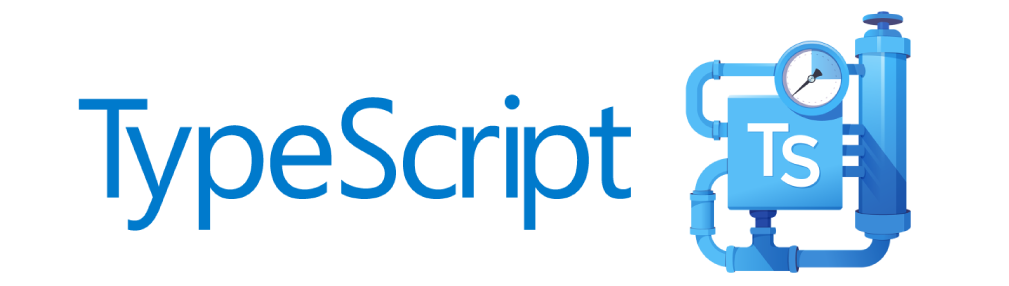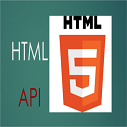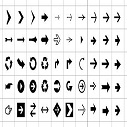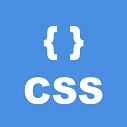# Persistent Interview Experience C++

Round 1: Online test for 60 minutes. Test consists of questions related to C++ and analytical problems.

Round 2: Technical interview

• What are the various OOPs concepts in C++ ?
• Difference between encapsulation and abstraction?
• What are the C++ access specifiers?
• How to call private function from child class ?
• What is the Diamond Problem in C++ ?
Answer : Check here and here
• What is Polymorphism and types of it ?
• Where and why use run-time Polymorphism ?
• Why use parameters int argc, char *argv[] in main function ?

• ```#include<iostream>
using namespace std;
#define main xyz
int main(int argc,char *argv[])
{
main();
return 0;
}
int xyz()
{
cout << "hi";
return 0;
}```
Will above program be compiled ? What will be the output ?

• ```struct s
{
char c;
int i;
};```
What is the size of the structure ?
• Write C++ program to print below output.
```      1
2 2
3 3 3
4 4 4 4
5 5 5 5 5
6 6 6 6 6 6 ```
The number of rows can be any.
```#include <iostream>

using namespace std;

int main()
{
int rows;int n;string space = " ";
cout << "Enter Number of rows" << endl;
cin >> rows;

cout << "rows : "<< rows << endl;
for (int t = 1; t <= rows; t++)
{
for (int i = t; i <= rows; i++)
{
cout << space;
}
n = t;
for(int j = 1; j <= t; j++)
{
cout << t;
if( n > 1 )
{
cout << space;
}
n--;
}
for (int k = t; k <= rows; k++)
{
cout << space;
}
cout << endl;
}

return 0;
}
```Ashwini Verma on Feb 05, 2020 at 12:05 am

#### Post Comment

##### WEB TECHNOLOGY##### TypeScript Programmning Language##### New HTML5 APIs##### HTML Arrow -> Range: Decimal 8592-8703. Hex 2190-21FF.##### How To Override One CSS Class With Another×